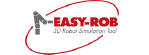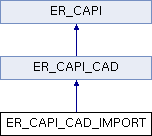EASY-ROB™ Application Programming Interface  v8.008

Method class for importing existing 3D geometries (IGP, STL, 3DS) More...

`#include <ER_CAPI.H>`## Static Public Member Functions

static ER_DllExport int Import_CAD (int cad_type=0, char *fln_name=0, char *body_name=0, float *scale=0)
Import a geometry file or create a primitive geometry to the current device group
`cad_type` can be one value of the following values.
CUBE_CAD_TYPE - Primitive Cube, scale[] = X, Y, Height
PYRAMID_CAD_TYPE - Primitive pyramide, scale[] = X, Y, Height, dx, dy
WEDGE_CAD_TYPE - Primitive wedge, scale[] = X, Y, Height X top, Y top, dx dy
CYLINDER_CAD_TYPE - Primitive cylinder, scale[] = Radius, Height 1, y Scale, Radius top, Height 2, y Scale top, dx, dy, Angle
CONE_CAD_TYPE - Primitive cone, scale[] = Radius, Height 1, y Scale, dx, dy, Angle
SPHERE_CAD_TYPE - Primitive sphere, scale[] = Radius, y Scale, Angle, beta 1, beta 2
IGP_CAD_TYPE - IGP file, scale[] = X,Y,Z
STL_CAD_TYPE - 3DS file, scale[] = X,Y,Z
_3DS_CAD_TYPE - 3DS file, scale[] = X,Y,Z
Remarks
... More...Static Public Member Functions inherited from ER_CAPI_CADStatic Public Attributes inherited from ER_CAPI_CAD
Method class for access to geometry parameter: Location, polygons, vertices, attributes, etc. More...

Method class for creating primitive geometries such as cubes, cylinder, spheres, etc. More...

Method class for importing existing 3D geometries (IGP, STL, 3DS) More...

Method class for converting external neutral and native CAD formats (STEP, IGES, JT-Open, CATIA, Pro/E, and so on) into the internal IGP Part File Format. More...Static Public Attributes inherited from ER_CAPI
static ER_CAPI_USER_IO er_capi_user_io
Method class for interaction with EASY-ROB. More...

static ER_CAPI_DEVICES er_capi_devices
Method class to create, attach, update devices, for kinematics calculations and for trajectory planning and -execution. More...

static ER_CAPI_SIM er_capi_sim
Method class for simulation settings. More...

static ER_CAPI_TARGETS er_capi_targets
Method class for paths and tags. More...

Method class for for 3D CAD Data import and -export, changing attributes and positions. More...

static ER_CAPI_SYS er_capi_sys
Method class for mathematical calculations, simulation status, units. More...

## Detailed Description

Method class for importing existing 3D geometries (IGP, STL, 3DS)

## Member Function Documentation

 static ER_DllExport int ER_CAPI_CAD_IMPORT::Import_CAD ( int cad_type = `0`, char * fln_name = `0`, char * body_name = `0`, float * scale = `0` )
static

Import a geometry file or create a primitive geometry to the current device group
`cad_type` can be one value of the following values.
CUBE_CAD_TYPE - Primitive Cube, scale[] = X, Y, Height
PYRAMID_CAD_TYPE - Primitive pyramide, scale[] = X, Y, Height, dx, dy
WEDGE_CAD_TYPE - Primitive wedge, scale[] = X, Y, Height X top, Y top, dx dy
CYLINDER_CAD_TYPE - Primitive cylinder, scale[] = Radius, Height 1, y Scale, Radius top, Height 2, y Scale top, dx, dy, Angle
CONE_CAD_TYPE - Primitive cone, scale[] = Radius, Height 1, y Scale, dx, dy, Angle
SPHERE_CAD_TYPE - Primitive sphere, scale[] = Radius, y Scale, Angle, beta 1, beta 2
IGP_CAD_TYPE - IGP file, scale[] = X,Y,Z
STL_CAD_TYPE - 3DS file, scale[] = X,Y,Z
_3DS_CAD_TYPE - 3DS file, scale[] = X,Y,Z
Remarks
...

Parameters
 [in] cad_type Type of geometry [in] fln_name file name of geometry [in] body_name name of geometry [in] scale Scaling vector depending on cad_type
Return values
 0 - ER_OK 1 - ER_ERROR

The documentation for this class was generated from the following file: